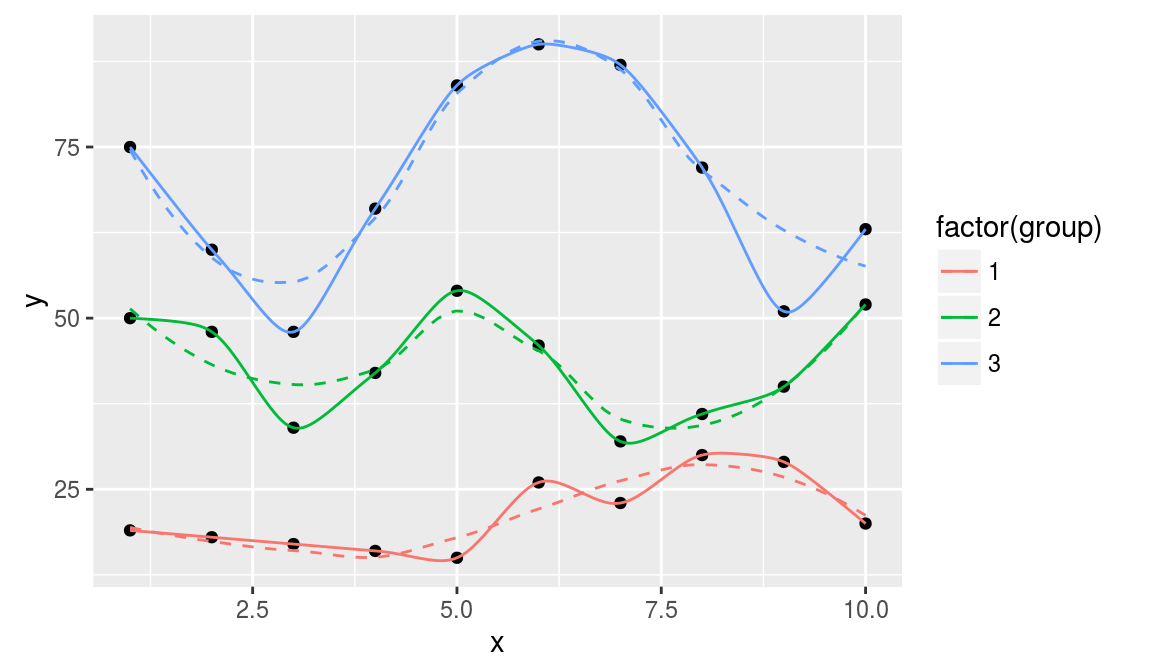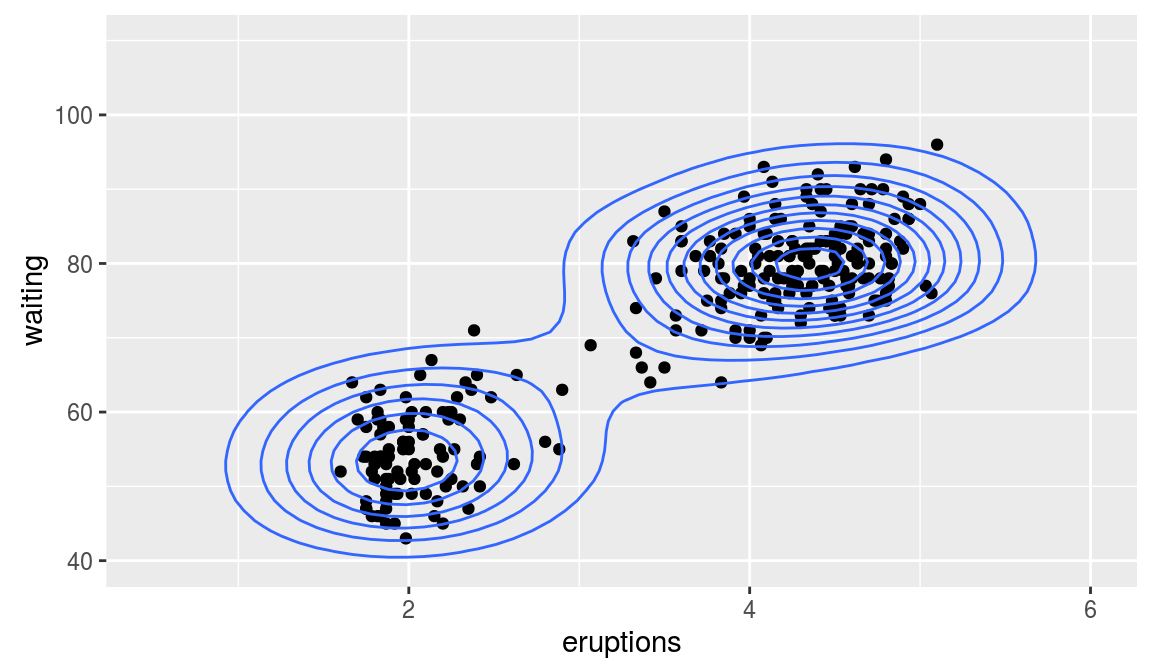ggplot2 now has an official extension mechanism. This means that others can now easily create their own stats, geoms and positions, and provide them in other packages. This should allow the ggplot2 community to flourish, even as less development work happens in ggplot2 itself. This page showcases these extensions.

### ggalt

https://github.com/hrbrmstr/ggalt

A compendium of ‘geoms’, ‘coords’ and ‘stats’ for ‘ggplot2’, including splines, 1d and 2d densities, univariate average shifted histograms and a new map coordinate system based on the ‘PROJ.4’-library..

``````# Example from https://github.com/hrbrmstr/ggalt
library(ggplot2)
library(gridExtra)
library(ggalt)

set.seed(1492)
dat <- data.frame(x=c(1:10, 1:10, 1:10),
y=c(sample(15:30, 10), 2*sample(15:30, 10), 3*sample(15:30, 10)),
group=factor(c(rep(1, 10), rep(2, 10), rep(3, 10)))
)

ggplot(dat, aes(x, y, group=group, color=factor(group))) +
geom_point(color="black") +
geom_smooth(se=FALSE, linetype="dashed", size=0.5) +
geom_xspline(spline_shape=-0.4, size=0.5)````````````## Alternate 2D density plots

ggplot(faithful, aes(x = eruptions, y = waiting)) +
geom_point() +
xlim(0.5, 6) +
ylim(40, 110) +
geom_bkde2d(bandwidth=c(0.5, 4))``````